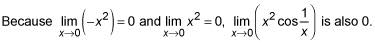##### Calculus Workbook For Dummies with Online PracticeThe sandwich or squeeze method is something you can try when you can’t solve a limit problem with algebra. The basic idea is to find one function that’s always greater than the limit function (at least near the arrow-number) and another function that’s always less than the limit function.

Both of your new functions must have the same limit as x approaches the arrow-number. Then, because the limit function is “sandwiched” between the other two, like salami between slices of bread, it must have that same limit as well.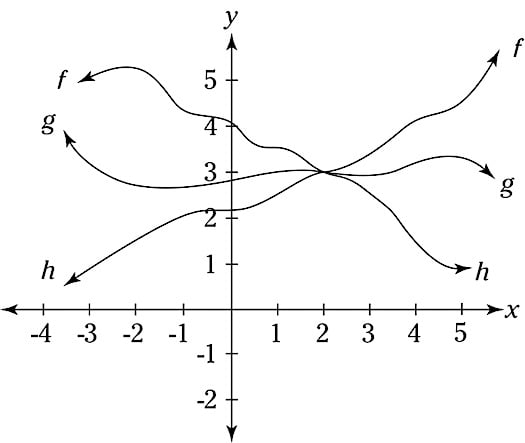A limit sandwich—functions f and h are the bread and g is the salami.

## Practice questions

1. Evaluate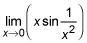2. Evaluate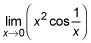1. The limit is 0. Here are three ways to do this. First, common sense should tell you that this limit equals 0.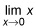is 0, of course, and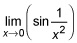never gets bigger than 1 or smaller than –1. You could say that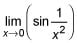therefore, is “bounded” (it’s bounded by –1 and 1). Then, because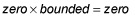the limit is 0. Don’t try this logic with you calc teacher—he won’t like it. Second, you can use your calculator: Store something small like 0.1 into x and then input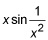into your home screen and hit enter. You should get a result of ~–0.05. Now store 0.01 into x and use the entry button to get back toand hit enter again. The result is ~0.003. Now try 0.001, then 0.0001 (giving you ~–0.00035 and ~0.00009), and so on. It’s pretty clear—though probably not to the satisfaction of your professor—that the limit is 0. The third way will definitely satisfy those typically persnickety professors. You’ve got to sandwich (or squeeze) your salami function,between two bread functions that have identical limits as x approaches the same arrow-number it approaches in the salami function. Because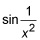never gets greater than 1 or less than –1,will never get greater than |x| or less than –|x|. (You need the absolute value bars, by the way, to take care of negative values of x.) This suggests that you can use b(x) = –|x| for the bottom piece of bread and t(x) = |x| as the top piece of bread. Graph b(x) = –|x|,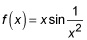and t(x) = |x| at the same time on your graphing calculator and you can see thatis always greater than or equal to –|x| and always less than or equal to |x|. Because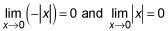and becauseis sandwiched between them,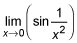must also be 0.
2. The limit is 0. Foruse b(x) = –x2 and t(x) = x2 for the bread functions. The cosine of anything is always between –1 and 1, so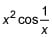is sandwiched between those two bread functions. (You should confirm this by looking at their graphs; use the following window on your graphing calculator—Radian mode, xMin = –0.15625, xMax = 0.15625, xScl = 0.05, yMin = –0.0125, yMax = 0.0125, yScl = 0.005.)